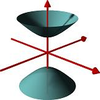#### You may also like### A Close Match

Can you massage the parameters of these curves to make them match as closely as possible?### Prime Counter

A short challenge concerning prime numbers.### The Right Volume

Can you rotate a curve to make a volume of 1?

# Numbered Graph

##### Age 16 to 18Challenge Level

We could experiment but let us try to be somewhat systematic.

Since one node will contain the number $11$ and must be connected to at least one other node, the node sum must be at least $12$.

The 'feet' now stand out as the four nodes only connected to one other node. Thus, from the numbers $1, \dots, 11$ we must create 4 pairs with the same sum of at least 12, without repeating any of the numbers.

We thus have the possibility of choosing four pairs from each of the following lines:

(sum 12) (11, 1), (10, 2), (9, 3), (8, 4), (7, 5) leaving (6, *, *) on the top row

(sum 13) (11, 2), (10, 3), (9, 4), (8, 5), (7, 6) leaving (1, *, *) on the top row

(sum 14) (11, 3), (10, 4), (9, 5), (8, 6) leaving (1, 2, 7) on the top row

(sum 15) (11, 4) (10, 5) (9, 6) (8, 7) leaving (1, 2, 3) on the top row

(sum > 15) Impossible to create four pairs.

Since the central node on the top row must have a node sum equal to the sum of the numbers in the top row we see that node sums of 14 and 15 are impossible, as the sum of the numbers in the top row would contradict this.

Furthermore, in the sum 12 case the sum of the two *s must equal 6. This is impossible, as each of the pairs we could use to fill the *s sum to 12.

Furthermore, in the sum 13 case, the sum of the two *s must equal 12. This is impossible, as each of the 5 possible pairs we could use to fill the *s sum to 13.

Therefore it is impossible to number the graph as required.